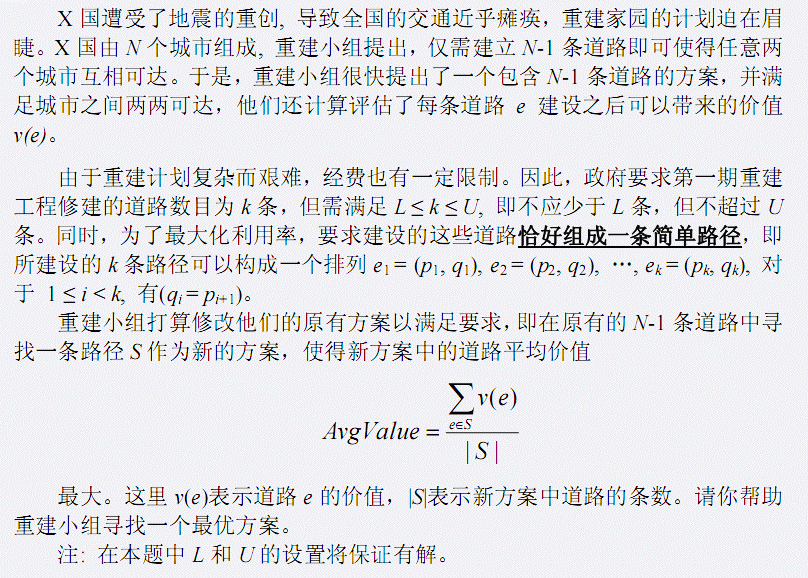# BZOJ1758 - [Wc2010]重建计划

BZOJ1758 - Click Here

# Description# Input

第一行包含一个正整数N，表示X国的城市个数。

第二行包含两个正整数L和U，表示政策要求的第一期重建方案中修建道路数的上下限。

接下来的N-1行描述重建小组的原有方案，每行三个正整数Ai,Bi,Vi分别表示道路(Ai,Bi)，其价值为Vi其中城市由1..N进行标号

# Output

输出最大平均估值，保留三位小数

# Solutions

毒瘤卡常

答案显然具有单调性。因此可以二分答案，这样就变成判断是否存在一条长度为$[L,U]$的路径，满足$0 \leq \sum_{e \in S} v(e) - AvgValue$。

对于该问题，可以考虑点分治解决。

计算一条经过$x$的路径最大值时，为了避免出现不合法情况，需要一棵子树一棵子树进行处理。

对于一个当前正在处理的子树，用$f(i)$表示该子树深度为$i$的路径的最大值，$g(i)$表示之前的子树深度为$i$的最大值。那么当前子树对答案的影响是$f(x) + max_{L - x \leq y \leq U - x} \, g(y)$，其中$x$是枚举当前子树的深度。同时容易发现，当$x$变化时，$[L - x , U - x]$是一个滑动的区间，因此$max$的部分也可以用单调队列维护。

接着来考虑一下时间复杂度。由于$g(y)$的值与之前子树的深度有关，因此一次更新答案的时间复杂度是当前已处理的子树深度的最大值。为了保证这个更新答案也是严格与子树大小有关，在进行子树操作前，需要对子树按照深度排序。

时间复杂度$O(n \log n \log V)$

然后就TLE了

因为人傻自带大常数，按照点分的模板去写会被T掉一个点

为了能够减小常数，在二分前需要预处理出点分树；在处理答案时，能break就break，然后就能卡过这题了…

# CODE

CODE - Click Here